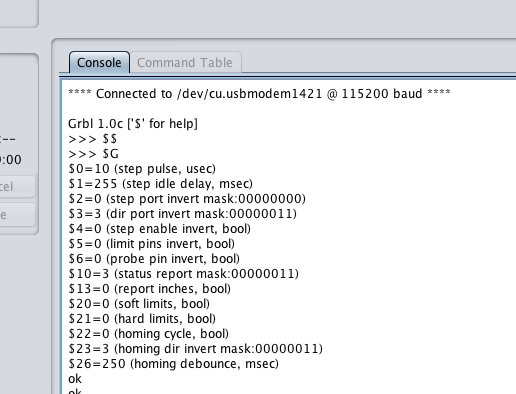# Universal GCode sender not configurable by axis

I am currently able to step my new XCarve with universal gcode sender and GRBL running on an arduino. My issue is that for a 10mm command, the XCarve moves about 30mm (Which I would like to fix via ugs), and ugs only displays these settings on \$\$ (Absent are x,y, or z steps/mm):
\$0=10 (step pulse, usec)
\$1=25 (step idle delay, msec)
\$2=0 (step port invert mask:00000000)
\$3=0 (dir port invert mask:00000000)
\$4=0 (step enable invert, bool)
\$5=0 (limit pins invert, bool)
\$6=0 (probe pin invert, bool)
\$10=3 (status report mask:00000011)
\$13=0 (report inches, bool)
\$20=0 (soft limits, bool)
\$21=0 (hard limits, bool)
\$22=0 (homing cycle, bool)
\$23=0 (homing dir invert mask:00000000)
\$26=250 (homing debounce, msec)

Is this a bug with the 2.0 nightly build of ugs or is there something I’m doing wrong? (More likely haha)

Thank you all.

Try changing your G91 (incremental) to a G90 (Absolute) and adding a decimal to the end of the 10. See if that helps. If not, then it’s probably a hardware adjustment…

@AnsonHaniwalt

Which version of grbl are you running? (version number should show when you connect to the serial port in UGCS).

1 Like

I had something like that on one of the boards I got from another vendor. For me it was something in the version of GRBL on the board. I ended up reflashing the board with the Inventables GRBL fork, and it fixed things right up.

https://inventables.desk.com/customer/portal/articles/2069033-grbl

@BradT, I took your advice and used the inventables fork, and @LarryM it now says that I am on GRBL version 1.0c, and I still have the list of limited commands. @cncrockstar I have no idea where the rest of the \$\$ readout went haha.I should probably mention that I’m using third party stepper controllers (TB6560s) with 1/16 microstepping, and now the machine is moving about 5mm for a 10mm command, which would be fixed by setting the default 1/8 microstepping to 1/16 microstepping in GRBL, however the problem seems to be that none of my changes (I’m changing the c code in the background and re-uploading to the arduino) seem to be taking effect. This is probably related to how I’m not seeing those setting on the \$\$ readout in ugs.

There is something wrong with the firmware you are using. This is what you should see if you have a 500mm X-Carve. If you have the 1000mm the only difference would be \$130 and \$131.

Unless you have a specific reason for doing so you do not need to change the source code. You may have messed something up in there.

Grbl 1.0c [’\$’ for help]

\$\$
\$0=10 (step pulse, usec)
\$1=255 (step idle delay, msec)
\$2=0 (step port invert mask:00000000)
\$3=3 (dir port invert mask:00000011)
\$4=0 (step enable invert, bool)
\$5=0 (limit pins invert, bool)
\$6=0 (probe pin invert, bool)
\$10=3 (status report mask:00000011)
\$11=0.020 (junction deviation, mm)
\$12=0.002 (arc tolerance, mm)
\$13=0 (report inches, bool)
\$20=0 (soft limits, bool)
\$21=0 (hard limits, bool)
\$22=0 (homing cycle, bool)
\$23=3 (homing dir invert mask:00000011)
\$24=25.000 (homing feed, mm/min)
\$25=750.000 (homing seek, mm/min)
\$26=250 (homing debounce, msec)
\$27=1.000 (homing pull-off, mm)
\$30=30000. (rpm max)
\$31=10000. (rpm min)
\$100=40.000 (x, step/mm)
\$101=40.000 (y, step/mm)
\$102=188.947 (z, step/mm)
\$110=8000.000 (x max rate, mm/min)
\$111=8000.000 (y max rate, mm/min)
\$112=500.000 (z max rate, mm/min)
\$120=500.000 (x accel, mm/sec^2)
\$121=500.000 (y accel, mm/sec^2)
\$122=50.000 (z accel, mm/sec^2)
\$130=290.000 (x max travel, mm)
\$131=290.000 (y max travel, mm)
\$132=100.000 (z max travel, mm)
ok

Could it be an issue with the EEPROM on my Arduino?

Here: https://github.com/grbl/grbl/wiki/Frequently-Asked-Questions it says that the GRBL settings list is stored in the EEPROM, that sometimes settings and parameters are funky after flashing GRBL, and that the EEPROM reset could be the fix for that; I’ll try it later tonight when I get home.

Try the command \$RST=\$ in the UGCS command tab.

\$RST=\$ : Erases and restores the \$\$ Grbl settings back to defaults